JEE  >  BITSAT Maths Test - 1

# BITSAT Maths Test - 1

Test Description

## 45 Questions MCQ Test BITSAT Mock Tests Series & Past Year Papers | BITSAT Maths Test - 1

BITSAT Maths Test - 1 for JEE 2023 is part of BITSAT Mock Tests Series & Past Year Papers preparation. The BITSAT Maths Test - 1 questions and answers have been prepared according to the JEE exam syllabus.The BITSAT Maths Test - 1 MCQs are made for JEE 2023 Exam. Find important definitions, questions, notes, meanings, examples, exercises, MCQs and online tests for BITSAT Maths Test - 1 below.
Solutions of BITSAT Maths Test - 1 questions in English are available as part of our BITSAT Mock Tests Series & Past Year Papers for JEE & BITSAT Maths Test - 1 solutions in Hindi for BITSAT Mock Tests Series & Past Year Papers course. Download more important topics, notes, lectures and mock test series for JEE Exam by signing up for free. Attempt BITSAT Maths Test - 1 | 45 questions in 60 minutes | Mock test for JEE preparation | Free important questions MCQ to study BITSAT Mock Tests Series & Past Year Papers for JEE Exam | Download free PDF with solutions
 1 Crore+ students have signed up on EduRev. Have you?
BITSAT Maths Test - 1 - Question 1

### The area enclosed between the ellipse x2 + 9y2 = 9 and the straight line x + 3y = 3, in the first quadrant is

Detailed Solution for BITSAT Maths Test - 1 - Question 1

The intersection points of x2 + 9y2 = 9  and x + 3y = 3 are (0,1) and (3,0).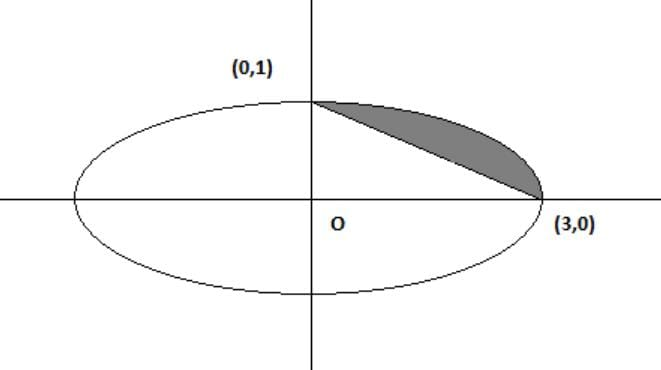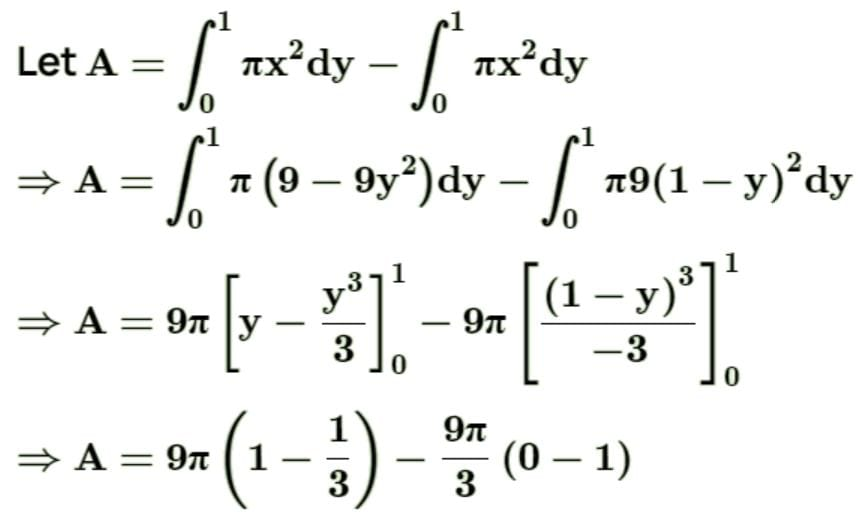∴ A = 6π − 3π = 3π.

BITSAT Maths Test - 1 - Question 2

### The coefficient of x-9 in the expansion of ((x2/2) - (2/x))9 is

Detailed Solution for BITSAT Maths Test - 1 - Question 2

The term x-9 will be T(10). Therefore T(10) will be
⇒ (-2/x)9
⇒ -512/x9
⇒ -512x-9

BITSAT Maths Test - 1 - Question 3

### The differential equation of the family of lines through the origin is

Detailed Solution for BITSAT Maths Test - 1 - Question 3

Let y = mx be the family of lines through origin. Therefore, dy/dx = m
Eliminating m, we get y = (dy/dx).x or x(dy/dx) – y = 0.

BITSAT Maths Test - 1 - Question 4

The circles x2 + y2 - 12x -12y = 0 and x2 + y2 + 6x + 6y = 0

Detailed Solution for BITSAT Maths Test - 1 - Question 4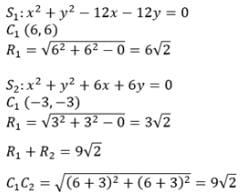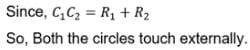BITSAT Maths Test - 1 - Question 5

Length of tangent drawn from (5,1) to the circle x+ y+ 6x - 4y - 3 = 0 is

Detailed Solution for BITSAT Maths Test - 1 - Question 5

Given circle is  x+ y+ 6x - 4y - 3 = 0 .....(i)
Given point is (5, 1) Let P = (5,1)
Now length of the tangent from P(x, y) to circle (i) = √ x+ y+ 2gx + 2fy + c​.
Now length of the tangent from P(5, 1) to circle (i) = √ 52+12+ 6.5 − 4.1 − 3​ = 7

BITSAT Maths Test - 1 - Question 6

The solution of differential equation (dy/dx) = [(x(2logx + 1)) / (siny + ycosy)] is

Detailed Solution for BITSAT Maths Test - 1 - Question 6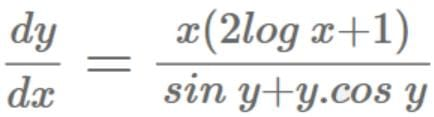(siny+ y.cosy)dy = [x(2logx + 1)]dx

Integrating both sides, we get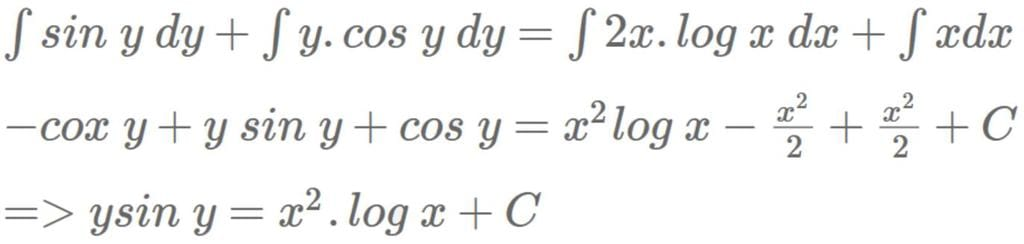BITSAT Maths Test - 1 - Question 7

The differential equation y(dy/dx) + x = c represent

Detailed Solution for BITSAT Maths Test - 1 - Question 7

ydy = (c−x)dx
Integrating both sides,
∫ydy = ∫(c−x)dx
y2​/2 = cx − x2​/2 + k
x2/2 + y2/2 ​− cx = k
x2 + y2 − 2cx = 2k
(x−c)2 + y2 = 2k + c2
This is equation of a circle with center on x-axis.

BITSAT Maths Test - 1 - Question 8

In an Argand plane the inequality π/4 < arg (z) < π/3 represent the region bounded by

Detailed Solution for BITSAT Maths Test - 1 - Question 8

We have π/4 ​< arg (z) < π/3​
Let z = x + iy
∴ arg (z) = tan-1(y/x​)
The given inequality can be written as
π/4 ​< tan-1(y/x​) < π/3​
⇒ tan(π/4) ​< y/x ​< tan(π/3)​
⇒ 1 < y/x ​< √3​
⇒ x < y < √3​x
This inequality represents the region between the lines
y = x and y = √3​x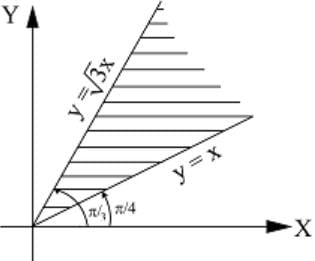BITSAT Maths Test - 1 - Question 9

(d/dx){log(secx+tanx)}=

Detailed Solution for BITSAT Maths Test - 1 - Question 9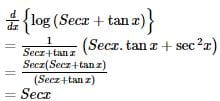BITSAT Maths Test - 1 - Question 10

If y =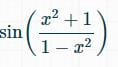, find dy/dx

BITSAT Maths Test - 1 - Question 11

9 + 16/2! + 27/3! + 42/4! + ..... ∞ is

BITSAT Maths Test - 1 - Question 12

The product of the perpendicular, drawn from any point on a hyperbola to its asymptotes is

BITSAT Maths Test - 1 - Question 13

From the top of the house 15 metres high the angle of depression of a point which is at a distance 15 m from the base of the house is

Detailed Solution for BITSAT Maths Test - 1 - Question 13

Given, Perpendicular = 15 m
Base = 15 m
Angle of depression = ​tanθ = 15/15 = 1
θ = 45°

BITSAT Maths Test - 1 - Question 14

The parabolas y2 = 4x and x2 = 4y divide the square region bounded by the lines x = 4, y = 4 and the coordinate axes. If S₁, S₂, S₃ are respectively the areas of these parts numbered from top to bottom; then S₁ : S₂ : S₃ is

Detailed Solution for BITSAT Maths Test - 1 - Question 14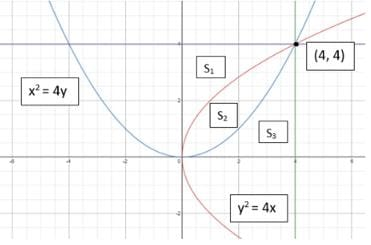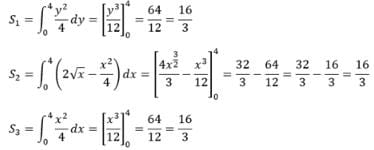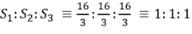BITSAT Maths Test - 1 - Question 15

The solution of the differential equation cos x sin y dx + sin x cos y dy = 0 is

Detailed Solution for BITSAT Maths Test - 1 - Question 15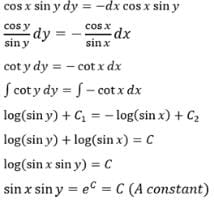BITSAT Maths Test - 1 - Question 16

4 tan⁻11/5 - tan⁻1 1/239 is equal to

Detailed Solution for BITSAT Maths Test - 1 - Question 16BITSAT Maths Test - 1 - Question 17

The period of the function sin(πx/2)+cos(πx/2) is

BITSAT Maths Test - 1 - Question 18

If A and B are any 2 x 2 matrics, then |A + B| = 0 implies

Detailed Solution for BITSAT Maths Test - 1 - Question 18

|A+B| = 0
⇒ A + B = O, where O is a zero matrix.
⇒ A = O − B = −B
Take determinant on both sides
⇒ |A| = −|B|
⇒ |A| + |B| = 0

BITSAT Maths Test - 1 - Question 19

A and B are square matrices of order n x n, then (A - B)2 is equal to

Detailed Solution for BITSAT Maths Test - 1 - Question 19

(A - B)2
⇒ (A - B) x (A - B)
⇒ (A - B) x A - (A - B) x B
⇒  A2 - AB - BA + B2

BITSAT Maths Test - 1 - Question 20

Let f(x) = x3 + 3x2 + 3x + 2. Then, at x = -1

Detailed Solution for BITSAT Maths Test - 1 - Question 20

f(x) = (x+1)3 + 1
∴ f'(x) = 3(x+1)2
f'(x) = 0  ⇒  x = -1
Now, f" (-1 - ∈) = 3(-∈)2 > 0, f'(-1 + ∈)2 = 3∈2 > 0
∴ f(x) has neither a maximum nor a minimum at x = -1
Let f'(x) = φ ′ (x) = 3(x+1)2
∴ φ′(x) = 6(x+1).
φ′(x) = 0  ⇒  x = -1
φ ′ (-1-∈) = 6(-∈) < 0, φ ′ (-1-∈) = 6∈ > 0
∴ φ (x) has a minimum at x = -1

BITSAT Maths Test - 1 - Question 21

If n ∈ N then n3 + 2n is divisible by

Detailed Solution for BITSAT Maths Test - 1 - Question 21

f(n) = n3 + 2n
put n=1, to obtain f(1) = 13 + 2.1 = 3
Therefore, f(1) is divisible by 3
Assume that for n=k, f(k) = k3 + 2k is divisible by 3
Now, f(k+1) = (k+1)3 + 2(k+1)
⇒ k3 + 2k + 3(k2 + k + 1)
⇒ f(k) + 3(k+ k + 1)
Since, f(k) is divisible by 3
Therefore, f(k+1) is divisible by 3
and from the principle of mathematical induction f(n) is divisible by 3 for all n∈N

BITSAT Maths Test - 1 - Question 22

If equation λx+ 2y- 5xy + 5x - 7y + 3 = 0, represents two straight lines, the value of λ is

BITSAT Maths Test - 1 - Question 23

If α + i β = tan⁻1 z, z = x + yi and α is constant, then locus of \'z\' is

BITSAT Maths Test - 1 - Question 24

The locus of the point of intersection of two normals to the parabola x2=8y, which are at right angles to each other,is

Detailed Solution for BITSAT Maths Test - 1 - Question 24

Let P(4t1, 2t12) and Q(4t2, 2t22) be the points on x2 = 8y. Equation of the normals at P and Q are
y - 2t12 = -1/t1(x - 4t12)  ....(1)
y - 2t22 = -1/t2 (x - 4t22)   ....(2)
Since the normals are at right angles, we have
(-1/t1)(-1/t2) = -1
⇒ t1t2 = -1   ....(3)
Solving Eqs. (1) and (2) and from Eq. (3), we have⇒ 2y = x2 + 12  which is the required locus.

BITSAT Maths Test - 1 - Question 25

The equation of the parabola with its vertex at (1,1) and focus at (3,1) is

Detailed Solution for BITSAT Maths Test - 1 - Question 25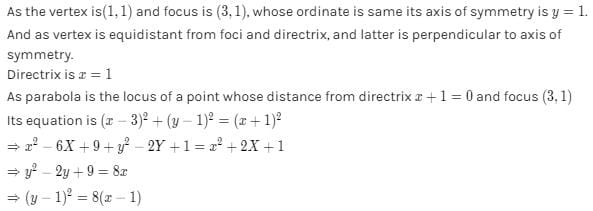BITSAT Maths Test - 1 - Question 26

Out of 6 boys and 4 girls a group of 7 is to be formed. In how many ways can this be done if the group is to have a majority of boys?

Detailed Solution for BITSAT Maths Test - 1 - Question 26

The boys are in majority, if groups formed are 4B 3G, 5B 2G, 6B 1G.
Total number of such combinations
6C4 x 4C3 + 6C5 x 4C2 + 6C6 x 4C1
⇒ 15 x 4 + 6 x 6 + 1 x 4
⇒ 60 + 36 + 4
⇒ 100

BITSAT Maths Test - 1 - Question 27

How many even numbers can be formed by using all the digits 2, 3, 4, 5, 6?

Detailed Solution for BITSAT Maths Test - 1 - Question 27

For a number to be even its last digit should be divisible by 2.
Therefore, only 2,4,6 can be used as last digits.
Number of such combinations,
⇒ 4!×3 = 24×3 = 72

BITSAT Maths Test - 1 - Question 28

A committee of five is to be chosen from a group of 9 people. The probability that a certain married couple will either serve together or not at all is

Detailed Solution for BITSAT Maths Test - 1 - Question 28

The total number of ways in which 5 person can be chosen out of 9 person is 9C5 = 126
The couple serves the committee in 7C3 x 2C2 = 35 ways
The couple does not serve the committee in
7C5 - 7C2 = 21 ways
Since the couple will either be together or not at all
∴ favourable number of cases = 35 + 21 = 56
∴ required porbability = 56 126 = 4/9

BITSAT Maths Test - 1 - Question 29

A six-faced dice is so biased that it is twice as likely to show an even number as an odd number when thrown. It is thrown twice. The probability that the sum of two numbers thrown is even is

Detailed Solution for BITSAT Maths Test - 1 - Question 29

∵ probability for odd = p

∴ probability for even = 2p

∵ p + 2p = 1

⇒ 3p = 1

⇒ p = 1/3​

∴ probability for odd = 1/3​ , probability for even = 2/3​

Sum of two no. is even means either both are odd or both are even

∴ required probability = (1/3​ × 1/3)​ + (2/3 ​× 2/3) ​= 1/9 ​+ 4/9 ​= 5/9​

BITSAT Maths Test - 1 - Question 30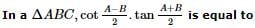Detailed Solution for BITSAT Maths Test - 1 - Question 30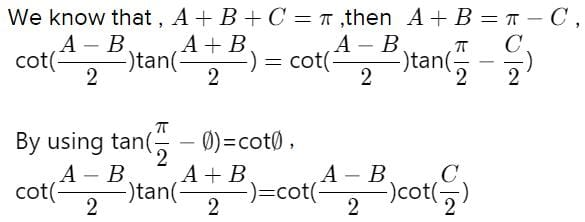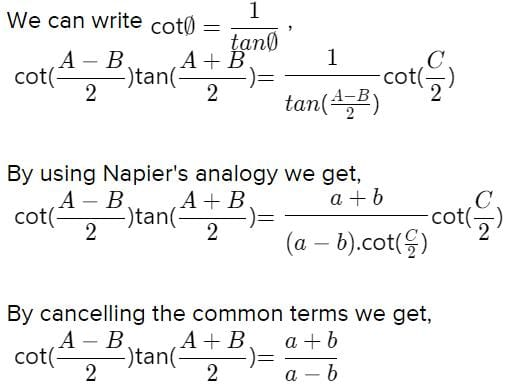BITSAT Maths Test - 1 - Question 31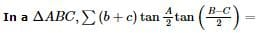Detailed Solution for BITSAT Maths Test - 1 - Question 31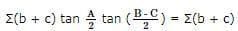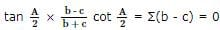BITSAT Maths Test - 1 - Question 32

The sum to infinity of (1/7) + (2/72) + (1/73) + (2/74) + ... is

Detailed Solution for BITSAT Maths Test - 1 - Question 32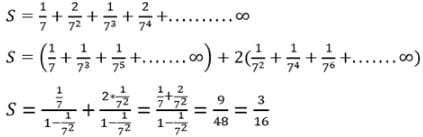BITSAT Maths Test - 1 - Question 33

If a, b are the roots of x2 + x + 1 = 0 then a2 + b2 =

Detailed Solution for BITSAT Maths Test - 1 - Question 33

Sum of the roots ⇒ a+b = −1                ...(1)
Product of the roots ⇒ ab = 1               ...(2)
We know that,
(a+b)= a+ b+ 2ab
⇒ a+ b= (a+b)− 2ab
Substituting (1) and (2) in the above equation, we get
⇒ a+ b= (−1)− 2(1)
∴ a+ b= −1

BITSAT Maths Test - 1 - Question 34

The third term of a G.P. is 3. The product of its first five terms is

Detailed Solution for BITSAT Maths Test - 1 - Question 34

Third term of G.P. = ar= 3

General term = arn−1

Product of first five terms = a5⋅r0+1+2+3+4

= a5r10=(ar2)5

= (3)5

= 243.

BITSAT Maths Test - 1 - Question 35

Suppose that g (x) = 1 + √x and f (g(x)) = 3 + 2 √x + x, then f (x) is

Detailed Solution for BITSAT Maths Test - 1 - Question 35

Given, g(x) = 1 + √x​

And f(g(x)) = 3 + 2√x​ + x

Now, f(g(x)) = 3 + 2√x​ + x [ terms splitting]

f(g(x)) = 2 + (1 + 2√x​ + x)

Also, (1 + √x​)2 = 1 + x + 2√x​

⇒ f(g(x)) = 2 + (1 + √x​)2

⇒ f(x) = 2 + x2

f(x) = x2 + 2​

BITSAT Maths Test - 1 - Question 36

The slope of the tangent of the curve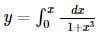at the point where x = 1 is

Detailed Solution for BITSAT Maths Test - 1 - Question 36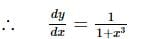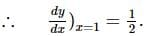BITSAT Maths Test - 1 - Question 37

If a line passes through points (4,3) and (2,λ) and perpendicular to y=2x+3, then λ=

Detailed Solution for BITSAT Maths Test - 1 - Question 37

Two lines are perpendicular then product of slope be -1

m1​m​= −1

(3 − λ)/2​ × 2 = −1

3 − λ = −1

3 + 1 = λ.

λ = 4.

BITSAT Maths Test - 1 - Question 38

The ratio in which the line joining the points (a,b,c) and (-a,-c,-b) is divided by the xy-plane is

Detailed Solution for BITSAT Maths Test - 1 - Question 38

Let λ:1 be the ratio in which the line joining the points (a,b,c) and (-a, -c, -b) is divided by xy- plane.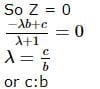BITSAT Maths Test - 1 - Question 39

The centre of sphere passing through four points (0, 0, 0), (0, 2, 0), (1, 0, 0) and (0, 0, 4) is

Detailed Solution for BITSAT Maths Test - 1 - Question 39

Since the sphere passes through origin, its equation is of the form
x+ y+ z+ 2ux + 2vy + 2wz = 0.  ...(1)
Substituting the co - ordinates of the other given points, we get
u = 1, v = 2, w = 4
Centre of sphere = (u/2, v/2, w/2)
⇒ (1/2, 1, 2)

BITSAT Maths Test - 1 - Question 40

The graph of y = logax is reflection of the graph of y = ax in the line

Detailed Solution for BITSAT Maths Test - 1 - Question 40

The graph of the logarithmic function y = logax is the reflection about the line y = x of the graph of the exponential function y = ax, as shown in Figure.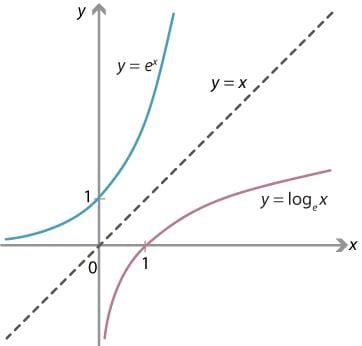BITSAT Maths Test - 1 - Question 41

The number of roots of the equation 2sin2θ + 3sinθ + 1 = 0 in 0,2π is

BITSAT Maths Test - 1 - Question 42

If sin[(π/4)cotθ] = cos[(π/4)tanθ], then θ=

Detailed Solution for BITSAT Maths Test - 1 - Question 42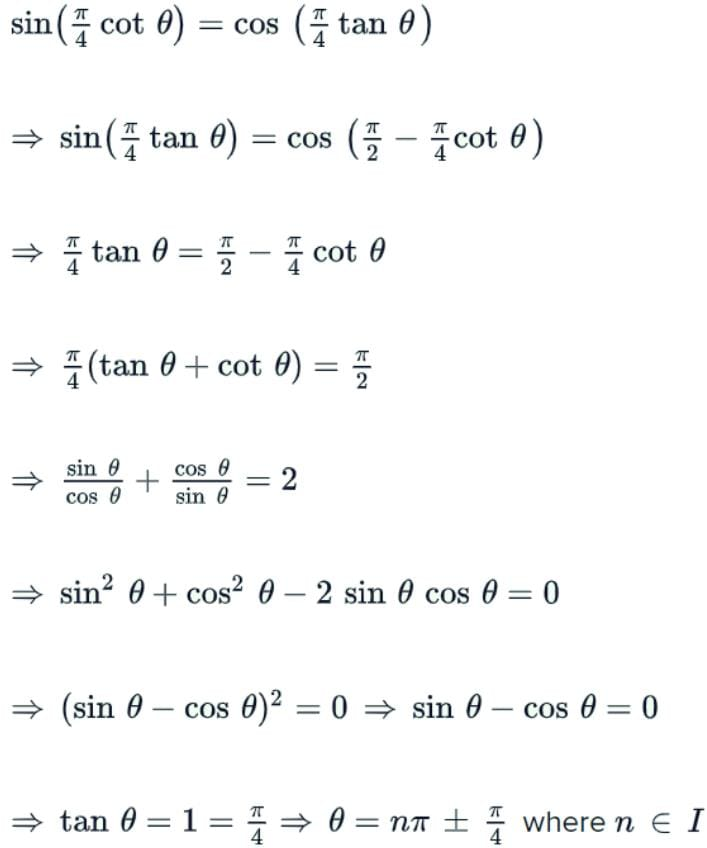BITSAT Maths Test - 1 - Question 43

If A+B+C=180o, then [(tanA+tanB+tanC)/(tanA tanB tanC)]=

Detailed Solution for BITSAT Maths Test - 1 - Question 43

Given, A + B + C = 180
So, A + B = 180 - C
Taking tan on both sides we get,
⇒ tan(A+B) = tan(180-C)
⇒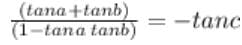⇒ tanA + tanB = -tanC(1 - tanA tanB)
⇒ tanA + tanB = - tanC + tanA tanB tanC
⇒ tanA + tanB + tanC = tanA tanB tanC

BITSAT Maths Test - 1 - Question 44

The points with position vectors 10î + 3ĵ, 12î - 5ĵ and aî + 11ĵ are collinear if a =

Detailed Solution for BITSAT Maths Test - 1 - Question 44

Given, A = (10i + 3j​)
B = (12i - 5j)​
C = (ai + 11j​)
AB = 2i - 8j​
AC = (a - 10)i + 8j​
AB and AC are collinear
⇒ 2/(a - 10) = -8/8
⇒ 2 = 10 - a
⇒ a = 8

BITSAT Maths Test - 1 - Question 45

a.b = 0, then

Detailed Solution for BITSAT Maths Test - 1 - Question 45

If a.b = 0
⇒ ab(cosθ) = 0
or cosθ = 0
⇒ θ = 90°
Therefore, a⊥b

## BITSAT Mock Tests Series & Past Year Papers

2 videos|15 docs|70 tests
 Use Code STAYHOME200 and get INR 200 additional OFF Use Coupon Code
Information about BITSAT Maths Test - 1 Page
In this test you can find the Exam questions for BITSAT Maths Test - 1 solved & explained in the simplest way possible. Besides giving Questions and answers for BITSAT Maths Test - 1, EduRev gives you an ample number of Online tests for practice

## BITSAT Mock Tests Series & Past Year Papers

2 videos|15 docs|70 tests